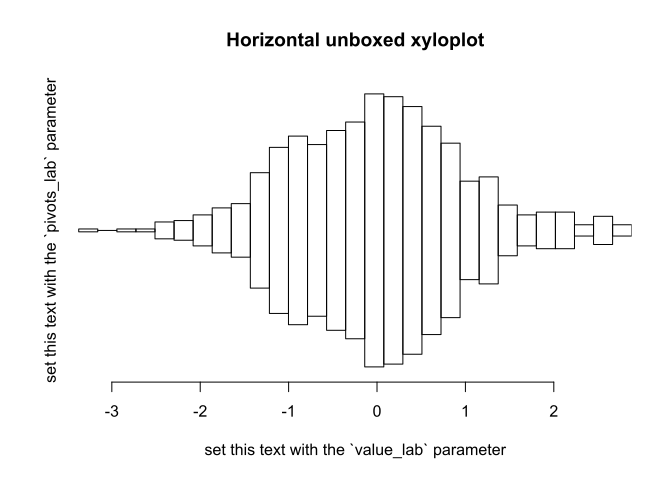# xyloplot examples

#### 2020-03-28

The xyloplot function displays continuous or discrete data as histogram style violin plots, or “xylophones”.

xyloplot accepts a simple numeric vector…

xyloplot(rnorm(1000))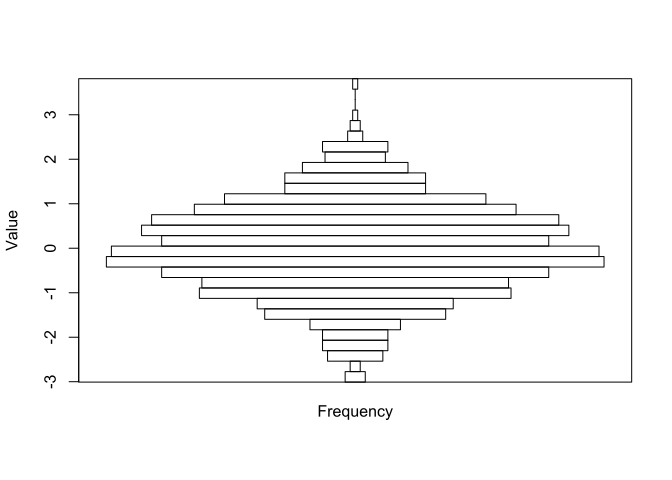… or a list of simple numeric vectors…

xyloplot(
x=lapply(1:3, function(mean) rnorm(mean=mean, n=1000)),
breaks=20,
col=rainbow(3))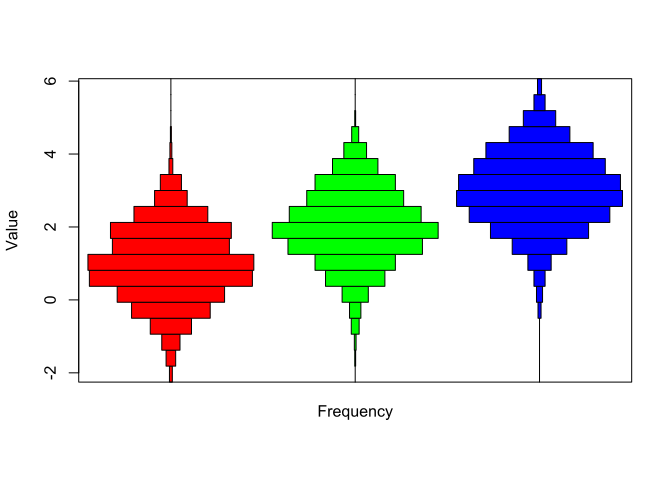… or discrete numeric data (supplied as a factor)…

values <- c(0.01, 0.1, 0.2, 0.25, 0.5, 1)
xyloplot(
replicate(n=5, simplify=FALSE,
expr=factor(sample(values, size=10, replace=TRUE), levels=values)),
col=rainbow(5))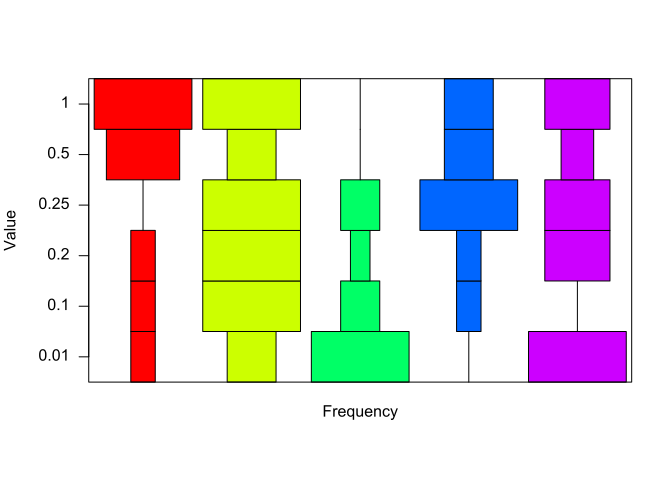… or general factors with wordy levels…

xyloplot(
sample(c("goldfish","cat","dog","fish","mouse","giraffe"),
size=100, replace=TRUE))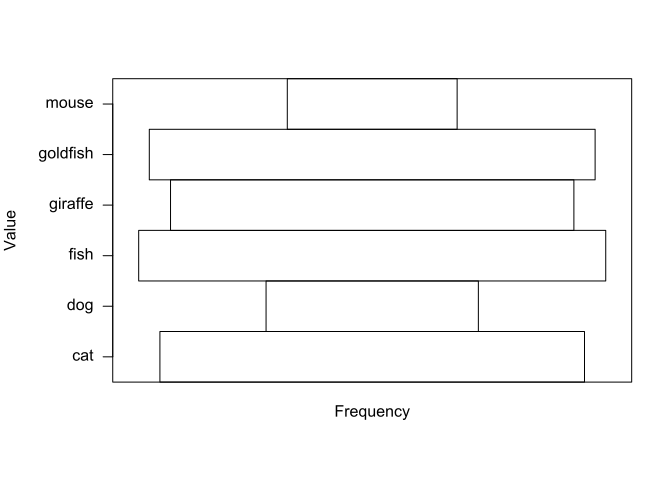The xylo_positions function gives you the x-coordinates of the centres of the xylophones in case you want to add further graphical objects. For example, here we’ll add the upper quartile as a red line for samples from 3 normal distributions.

data <- lapply(1:3, function(mean) rnorm(mean=mean, n=1000))
xyloplot(x=data)
n <- length(data)
positions <- xylo_positions(n)
upper_qs <- sapply(data, function(sample) quantile(sample, prob=0.75))
segments(x0=positions-1/n/2, x1=positions+1/n/2, y0=upper_qs, y1=upper_qs, col="red", pch=19)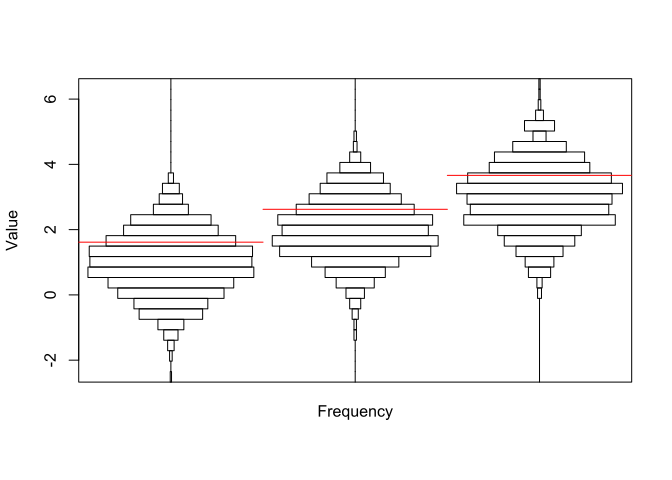xyloplot can be used to plot population demographics-style histograms (i.e. ‘split in the middle’) using the left_right_xylo function. This function must be supplied a left-hand side vector (or list of vectors), lhs, and a right-hand side vector (or list of vectors of the same length as lhs), rhs. A length-2 vector of colours can then be used to colour the left and right-hand sides respectively.

data_rhs <- lapply(2:4, function(mean) rnorm(mean=mean, sd=2, n=2000))
left_right_xylo(lhs=data, rhs=data_rhs, col=rainbow(2))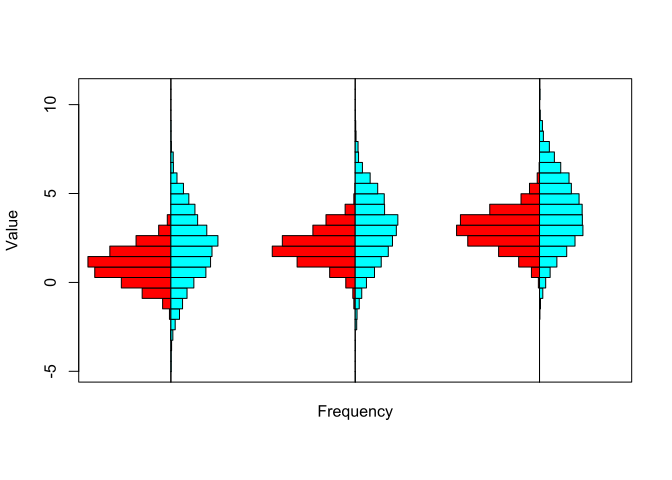xyloplot accepts a just argument, a vector whose elements correspond to the elements of the list x (and which should therefore be possible to recycle to the same length as x), and whose components should take values in {0, 0.5, 1}. Values of 0 cause the bars of the resultant xyloplot to align to the pivots on the left (like a traditional histogram), values of 0.5 cause them to align centre align, and values of 1 cause them to align to the right. Note that graphical options, e.g. col, are recycled over the distributions going from left to right, regardless of whether they are aligned to the centre, left or right.

xyloplot also accepts a pivot argument, which like just should be a vector whose elements correspond to the elements of x, and determine which pivot the histograms representing the distributions in each element of x should be assigned to.

Note that the left_right_xylo argument is thus a shorthand method of generating the equivalent plot with xyloplot

xyloplot(c(data, data_rhs), pivot=rep(1:3, 2), just=rep(1:0, each=3), col=rep(rainbow(2), each=3))

Multiple xylophones can be placed on the same pivot by including duplicated elements in the pivot argument. Of course they would overlap each other, however the extent of the overlap can be seen if using non-opaque colours.

xyloplot(list(rnorm(1000), rnorm(1000)+2), pivot=rep("a", 2), col=rainbow(n=2, alpha=0.5))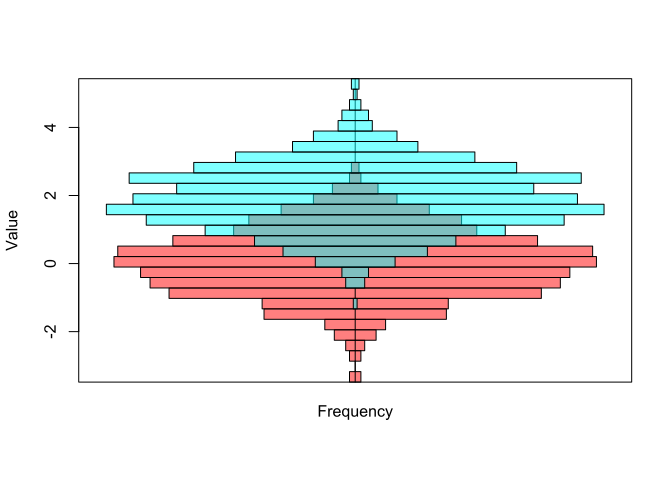xyloplot accepts a freq argument. It is equivalent to the freq argument in hist: a logical value which if FALSE (default) makes the width of bars represent frequency densities, and if TRUE, makes the width of bars represent frequencies/counts. In the example below, note that the rhs object contains twice as many observations as the lhs object. Setting freq to TRUE will therefore mean that the right-hand side bars collectively occupy twice as much width as the left-hand side ones.

lhs <- rnorm(n=1000)
rhs <- rnorm(n=2000)
plot(main="freq=FALSE", left_right_xylo(lhs=lhs, rhs=rhs, col=rainbow(2), freq=FALSE))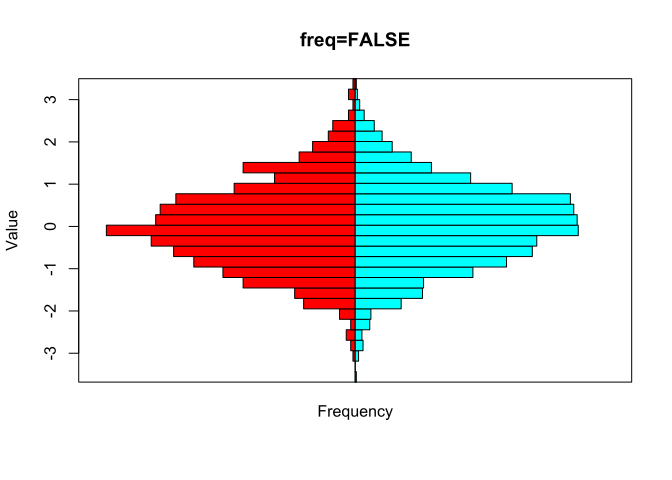plot(main="freq=TRUE", left_right_xylo(lhs=lhs, rhs=rhs, col=rainbow(2), freq=TRUE))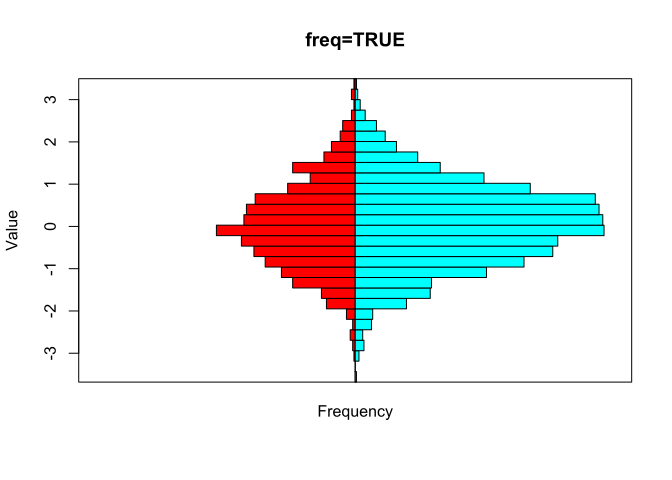## xyloplot objects

The xyloplot function returns an object of class "xyloplot", containing information about the graphical elements required to construct the plot. Plots with default parameters are generated when the object is printed. In order to set some graphical parameters, the S3 generic plot function for xyloplots must be called on the object.

Examples include creating horizontal xyloplots (vertical=FALSE), removing the box around the plot which is drawn by default (box=FALSE), and setting the labels for the x and y axis. For more options, see the help page ?plot.xyloplot.

plot(
main="Horizontal unboxed xyloplot",
vertical=FALSE,
box=FALSE,
pivots_lab="set this text with the pivots_lab parameter",
value_lab="set this text with the value_lab parameter",
x=xyloplot(x=rnorm(1000)))